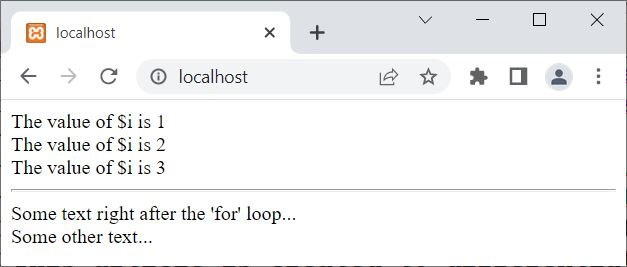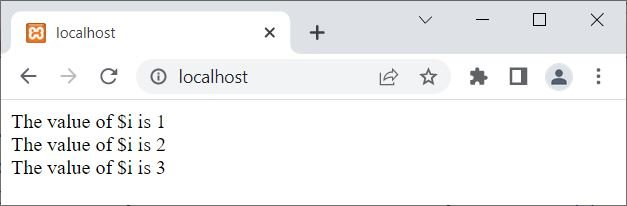# Difference between exit and break in PHP

The PHP exit() method stops the current PHP script from running. The break statement, on the other hand, stops the current loop or switch structure from running. For example, let me use break first:

```<?php
for(\$i=1; \$i<10; \$i++)
{
if(\$i==4)
break;
echo 'The value of \$i is ' . \$i . '<br>';
}
echo '<hr>';
echo 'Some text right after the "for" loop...<br>';
echo 'Some other text...<br>';
?>```

The following line of code initializes a variable \$i to 1, and sets the loop condition to continue while \$i is less than 10. On each iteration, the variable \$i is incremented using the post-increment operator \$i++.

`for(\$i=1; \$i<10; \$i++):`

And the following block of code

```if(\$i==4)
break;```

checks if the value of \$i is equal to 4, and if so, it breaks out of the loop using the break statement. In this case, since the loop condition is set to continue while \$i is less than 4, this if statement is unnecessary and will never be executed.

The next line of code prints the value of "\$i" to the screen, along with some extra text. The dot operator (.) concatenates the string "The value of \$i is" with the value of "\$i" and the "<br>" tag, which adds a line break.

`echo 'The value of \$i is ' . \$i . '<br>';`

The output of this PHP example is:And let me create the same example, using exit():

```<?php
for(\$i=1; \$i<10; \$i++)
{
if(\$i==4)
exit();
echo 'The value of \$i is ' . \$i . '<br>';
}
echo '<hr>';
echo 'Some text right after the "for" loop...<br>';
echo 'Some other text...<br>';
?>```

Similar to "<br>", the "<hr>" tag creates a horizontal line. Now the rest of the code is easily understandable based on the previous explanation.

Now in this example, since I used "exit()" (which stops the complete PHP scripts from execution) instead of "break" (which only breaks or stops the current loop's execution), the remaining PHP script or code will not be executed, even if the code is written outside the loop. Therefore, the output should be:Another thing I wanted to mention is that both exit() and exit can be used to end a script's execution. However, because exit() is a function and follows the standard function call syntax in PHP, it is considered to be the more "proper" way of doing so.

That is, the break is used when we need to exit from a loop, whereas the exit is used when we need to exit from the whole PHP script or program.

PHP Online Test

« Previous Tutorial Next Tutorial »